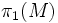# Bieberbach theorem

Let$M$ be a compact flat Riemannian manifold of dimension$n$. Then:
•$\pi_1(M)$ (the fundamental group) of$M$) contains a free Abelian normal subgroup of rank$n$ and finite index
• Thus$M$ is a finite quotient of a flat torus (using the fact that the only Riemannian manifolds whose fundamental groups are free Abelian, are the flat tori).